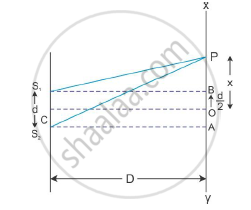# Derive an Expression for Path Difference in Young’S Double Slit Experiment - Physics

Derive an expression for path difference in Young’s double slit experiment and obtain the conditions for constructive and destructive interference at a point on the screen.

#### Solution

Diagrammatical arrangement for Young’s double slit experiment:In the above figure, S1 and S2 are two narrow closely spaced slits illuminated by monochromatic light of wavelength λ. The screen on which the interference pattern is observed is represented as XY.

If S1 and S2 emit light in the same phase, then for point O, the path difference receives light in the same phase. The superposition at O is constructive producing a bright point called the central maxima. The intensity at any point P at a distance x from O depends on the path difference between light reaching P from S1 and S2. The path difference is S2P − S1P.

From the geometry of the figure, we have

(S_2P)^2=D^2+(x+d/2)^2

Similarly, we have

(S_1P)^2=D^2+(x+d/2)^2

:.(S_2P)^2-(S_1P)^2=D^2+(x+d/2)^2-D^2-(x-d/2)^2

:.(S_2P)^2-(S_1P)^2=(x+d/2)^2-(x-d/2)^2

:.(S_2P)^2-(S_1P)^2=x^2+xd+d^2/4-x^2+xd-d^2/4

∴ (S2P)2-(S1P)2=2xd

∴(S2P-S1P)(S2P+S1P)=2xd

:.S_2P-S_1P=(2xd)/(S_2P+S_1P)

Now, from the figure we can see that

S2P≈S1P = D

:.S_2P-S_1P=(2xd)/(2D)=(xd)/D

This is the expression for path difference.

Condition for constructive interference: Constructive interference will occur when the phase difference between the two superposing waves is an even multiple of π or the path difference is an integral multiple of wavelength λ.

Condition for destructive interference: Destructive interference will occur when the phase difference between the two superposing waves is an odd multiple of π or the path difference is an odd multiple of wavelength λ/2.

Concept: Interference of Light Waves and Young’S Experiment
Is there an error in this question or solution?